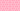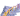PricingResources

# How to Calculate Standard Deviation

Written by

In the world of finance and investing, we often rely on statistical models. A good example is standard deviation, which offers a way to measure the volatility of any data set. By learning more about the standard deviation meaning and how it’s calculated, you can add it to your investing toolkit. Here’s how to get started.

## What is standard deviation?

Used in statistics, standard deviation provides a measure of how widely the points in a dataset swing back and forth from the mean. To find standard deviation, you calculate the square root of the set’s variance. When data points are further away from the mean, this indicates higher standard deviation.

The standard deviation meaning is often applied to an investment’s rate of return. The more that these data points deviate from the mean, the more volatility involved with the investment. Stable blue-chip stocks typically show a low standard deviation, while new, untested stocks will show higher standard deviation. For investors, this statistical measure offers a way to assess an investment’s level of risk.

## .css-g8fzsc{padding:0;margin:0;font-weight:700;}Understanding standard deviation vs. variance

Any standard deviation calculator takes variance into account, but what is the difference between these two terms? Standard deviation is calculated by taking the square root of variance. Variance shows the data’s spread in relation to its mean value. With more significant variation in data points, there will be a more considerable variance. Calculating standard deviation simply makes variance easier to interpret and visualize.

### The standard deviation formula

The standard deviation formula is based on a series of data points from a set. These are plugged into the following equation:

## How to calculate standard deviation

Perhaps the easiest way to find standard deviation is to use an online calculator. However, absent a standard deviation calculator, you can also work it out using the formula above:

1. Calculate the data set’s mean value by adding together its data points and dividing this value by the total number of points.

2. Figure out the variance for all data points. To do this, subtract the mean value from each data point value.

3. Square each variance and add the totals together to get a single figure. You can then divide this by the total number of data points, minus one.

4. Find standard deviation by calculating the square root of the variance you’ve just calculated.

## How to use standard deviation

Now that you’ve used the standard deviation calculator or formula, what can you do with these results? We’ve already touched on its use in investing above. This metric can be used to measure the volatility of a security, but you can also apply it to the market and even predict future trends. Growth funds will have a high standard deviation, while index funds will be lower since their aim is to track the index as closely as possible.

It’s easy to assume that low standard deviation is better for investors, since it indicates lower levels of risk. However, this isn’t always the case. Growth funds might be riskier but can lead to higher potential rates of return over time.

Standard deviation offers only one metric of volatility, but it does also come with certain limitations. For example, it assumes normal distribution and will look at all uncertainty as risk. An index might be showing higher-than-average returns, but because these are far away from the mean standard deviation would show the same level of risk as lower-than-average returns. As with any investment, look at the bigger picture before thinking about your appetite for risk and overall goals.

#### We can help

GoCardless helps you automate payment collection, cutting down on the amount of admin your team needs to deal with when chasing invoices. Find out how GoCardless can help you with ad hoc payments or recurring payments.## Interested in automating the way you get paid? GoCardless can help

Contact sales

Sales

Contact Sales

+1(415) 523-2279

Support

help@gocardless.com

+1 (628) 241-0044